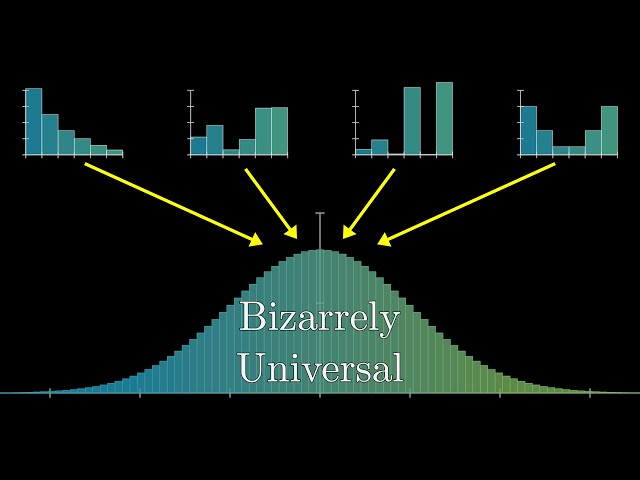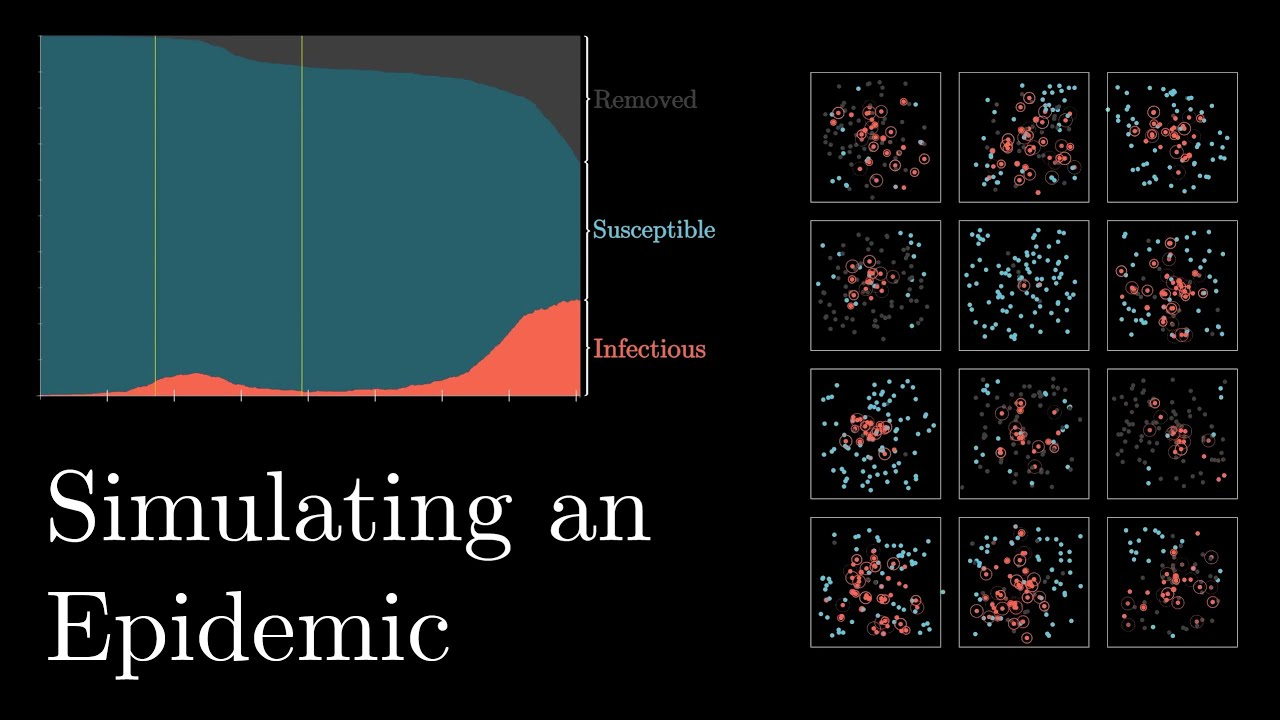# 3b1bMathematics Statistics

#### But what is the Central Limit Theorem?

3Blue1Brown provides a visual introduction to probability’s most important theorem.Mathematics

#### The mathematically optimal Wordle strategy

An excuse to teach a lesson on information theory and entropy.Data Visualization Neural Networks

#### Visualizing Deep Learning

vcubingx provides a visual introduction to the structure of an artificial neural network. The Neural Network, A Visual Introduction | Visualizing Deep Learning, Chapter 1 0:00 Intro 1:55 One input Perceptron 3:30 Two input Perceptron 4:40 Three input Perceptron 5:17 Activation Functions 6:58 Neural Network 9:45 Visualizing 2-2-2 Network 10:59 Visualizing 2-3-2 Network 12:33 Classification […]Mathematics

#### Why Do Prime Numbers Make These Spirals?

3Blue1Brown  explores a fascinating property of prime numbers.Mathematics Science

#### Simulating an Epidemic

3Blue1Brown walks through the mathematics of epidemics through simulations. The source code for this video is visible here: https://github.com/3b1b/manim/blob/shaders/from_3b1b/active/sir.py